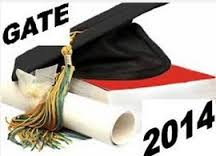## GATE Syllabus for Instrumentation Engineering (IN) 2014

GATE Syllabus for Instrumentation Engineering (IN) 2014 : 1. Instrumentation Engineering stream is provided only by some engineering colleges, so in order to gain better knowledge in this field candidates plan for their masters.2. M.Tech is one of the post graduation course which is taken by most of the instrumentation engineering students. All these candidates must appear for GATE exam to get admission in any college.

3. Look at the syllabus for Instrumentation Engineering (IN) in GATE-2014 exam

4. ENGINEERING MATHEMATICS

Linear Algebra: Matrix Algebra, Systems of linear equations, Eigen values and eigen vectors.

Calculus: Mean value theorems, Theorems of integral calculus, Evaluation of definite and improper integrals, Partial Derivatives, Maxima and minima, Multiple integrals, Fourier series. Vector identities, Directional derivatives, Line, Surface and Volume integrals, Stokes, Gauss and Green’s theorems.

Differential equations: First order equation (linear and nonlinear), Higher order linear differential equations with constant coefficients, Method of variation of parameters, Cauchy’s and Euler’s equations, Initial and boundary value problems, Partial Differential Equations and variable separable method.

GATE 2014 Online Exam Date

Complex variables: Analytic functions, Cauchy’s integral theorem and integral formula, Taylor’s and Laurent’ series, Residue theorem, solution integrals.

Probability and Statistics: Sampling theorems, Conditional probability, Mean, median, mode and standard deviation, Random variables, Discrete and continuous distributions, Poisson,Normal and Binomial distribution, Correlation and regression analysis.

Numerical Methods: Solutions of non-linear algebraic equations, single and multi-step methods for differential equations.

Transform Theory: Fourier transform,Laplace transform, Z-transform.

5. INSTRUMENTATION ENGINEERING
Basics of Circuits and Measurement Systems: Kirchoff’s laws, mesh and nodal Analysis. Circuit theorems. One-port and two-port Network Functions. Static and dynamic characteristics of Measurement Systems. Error and uncertainty analysis. Statistical analysis of data and curve fitting.

Transducers, Mechanical Measurement and Industrial Instrumentation: Resistive, Capacitive, Inductive and piezoelectric transducers and their signal conditioning. Measurement of displacement, velocity and acceleration (translational and rotational), force, torque, vibration and shock. Measurement of pressure, flow, temperature and liquid level.Measurement of pH, conductivity, viscosity and humidity.

GATE Syllabus for Mathematics 2014

Analog Electronics: Characteristics of diode, BJT, JFET and MOSFET. Diode circuits. Transistors at low and high frequencies, Amplifiers, single and multi-stage. Feedback amplifiers. Operational amplifiers, characteristics and circuit configurations. Instrumentation amplifier. Precision rectifier. V-to-I and I-to-V converter. Op-Amp based active filters. Oscillators and signal generators.

Digital Electronics: Combinational logic circuits, minimization of Boolean functions. IC families, TTL, MOS and CMOS. Arithmetic circuits. Comparators, Schmitt trigger, timers and mono-stable multi-vibrator. Sequential circuits, flip-flops, counters, shift registers. Multiplexer, S/H circuit.Analog-to-Digital and Digital-to-Analog converters. Basics of number system.Microprocessor applications, memory and input-output interfacing. Microcontrollers.

Signals, Systems and Communications: Periodic and aperiodic signals. Impulse response, transfer function and frequency response of first- and second order systems. Convolution, correlation and characteristics of linear time invariant systems. Discrete time system, impulse and frequency response. Pulse transfer function. IIR and FIR filters. Amplitude and frequency modulation and demodulation. Sampling theorem, pulse code modulation. Frequency and time division multiplexing. Amplitude shift keying, frequency shift keying and pulse shift keying for digital modulation.

Electrical and Electronic Measurements: Bridges and potentiometers, measurement of R,L and C. Measurements of voltage, current, power, power factor and energy. A.C & D.C current probes. Extension of instrument ranges. Q-meter and waveform analyzer. Digital voltmeter and multi-meter. Time, phase and frequency measurements. Cathode ray oscilloscope. Serial and parallel communication. Shielding and grounding.

Control Systems and Process Control: Feedback principles. Signal flow graphs. Transient Response, steady-state-errors. Routh and Nyquist criteria. Bode plot, root loci. Time delay systems. Phase and gain margin. State space representation of systems. Mechanical, hydraulic and pneumatic system components. Synchro pair, servo and step motors. On-off, cascade, P, P-I, P-I-D, feed forward and derivative controller, Fuzzy controllers.

Analytical, Optical and Biomedical Instrumentation: Mass spectrometry. UV, visible and IR spectrometry. X-ray and nuclear radiation measurements. Optical sources and detectors, LED, laser, Photo-diode, photo-resistor and their characteristics. Interferometers, applications in metrology. Basics of fiber optics. Biomedical instruments, EEG, ECG and EMG. Clinical measurements. Ultrasonic transducers and Ultrasonography. Principles of Computer Assisted Tomography.

6. Visit the official website of GATE-2014 for more details - http://gate.iitkgp.ac.in/gate2014/